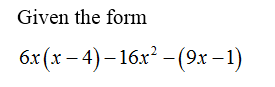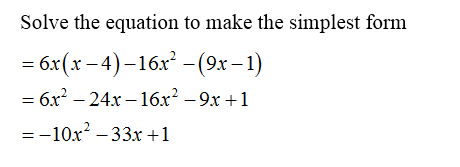# What is the simplest form of the expression 6x(x − 4) − 16x2 − (9x − 1)? A. -10x2 − 33x + 1B. 10x2 − 33x + 1C. -10x − 33x + 1D. -10x2 + 33x + 1

Question
10 views
What is the simplest form of the expression 6x(x − 4) − 16x2 − (9x − 1)?

A.
-10x2 − 33x + 1
B.
10x2 − 33x + 1
C.
-10x − 33x + 1
D.
-10x2 + 33x + 1
check_circle

Step 1Step 2...

### Want to see the full answer?

See Solution

#### Want to see this answer and more?

Solutions are written by subject experts who are available 24/7. Questions are typically answered within 1 hour.*

See Solution
*Response times may vary by subject and question.
Tagged in

### Algebra